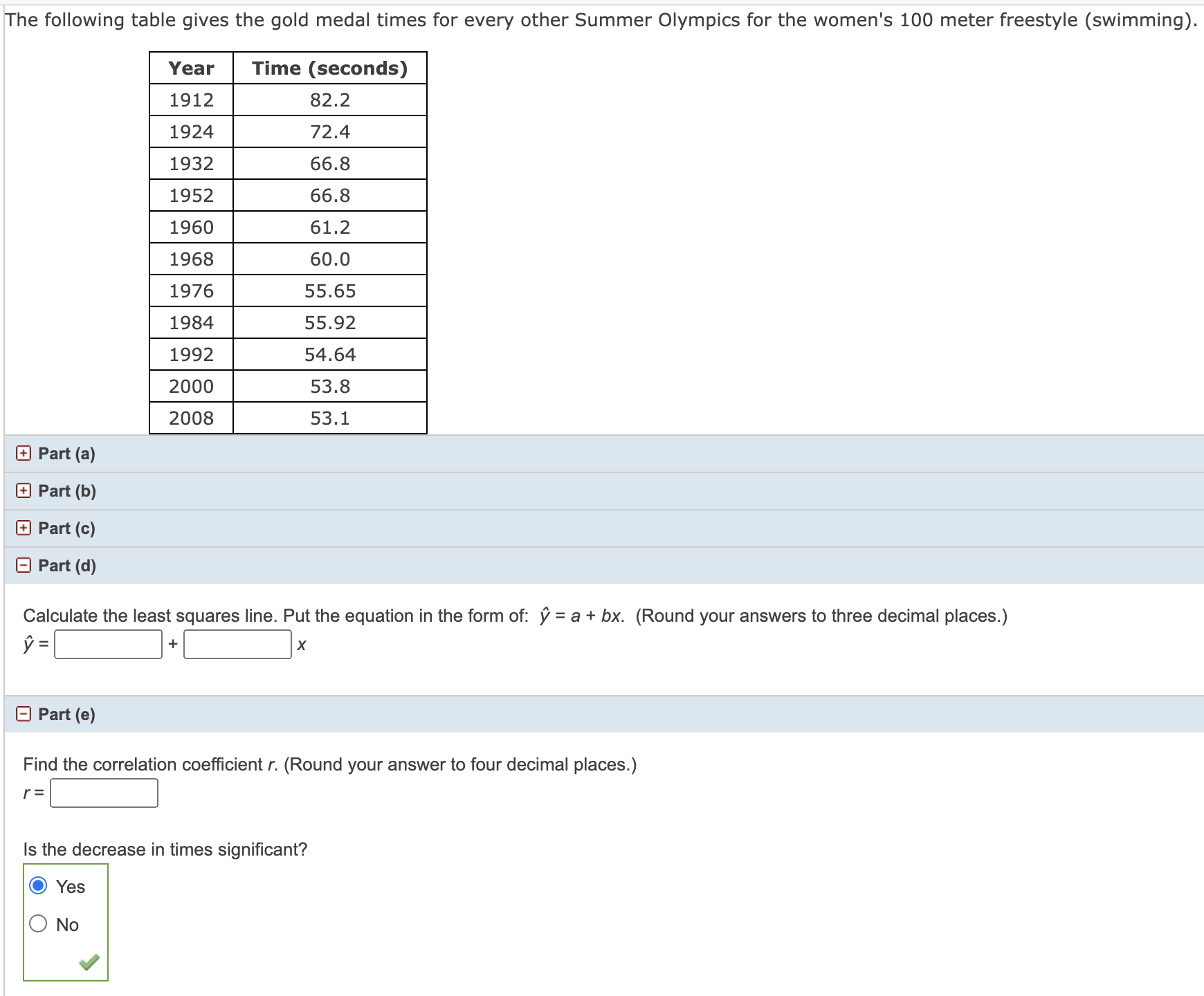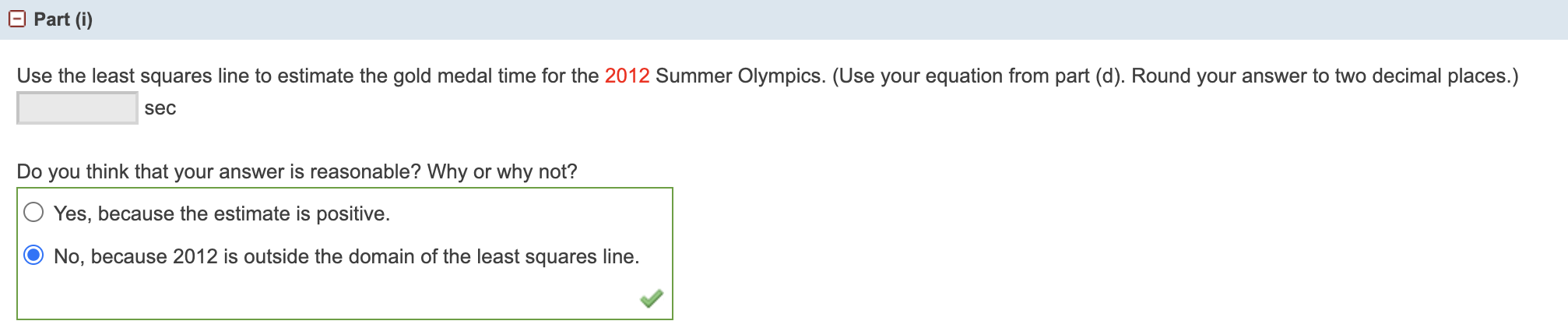Home / Expert Answers / Statistics and Probability / part-a-part-b-part-c-part-d-calculate-the-least-squares-line-put-the-equation-in-the-form-pa707

# (Solved): Part (a) Part (b) Part (c) Part (d) Calculate the least squares line. Put the equation in the form ...Part (a) Part (b) Part (c) Part (d) Calculate the least squares line. Put the equation in the form of: . (Round your answers to three decimal places.) Part (e) Find the correlation coefficient . (Round your answer to four decimal places.) Is the decrease in times significant? Yes No Use the least squares line to estimate the gold medal time for the 2012 Summer Olympics. (Use your equation from part (d). Round your answer to two decimal places.) Do you think that your answer is reasonable? Why or why not? Yes, because the estimate is positive. No, because 2012 is outside the domain of the least squares line.

We have an Answer from Expert